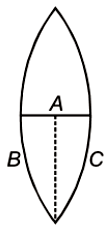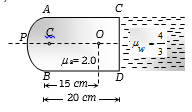You’ve reached the end of your free Videos limit.
#13 | Image Formed by Lens
(Physics) > Ray Optics and Optical Instruments
Unable to watch the video, please try another server
Related Practice Questions :

A concave lens of focal length 25 cm produces image $\frac{1}{10}th$ the size of the object. The distance of the object from the lens is

(1) 225 cm

(2) 250 cm

(3) 150 cm

(4) 175 cm

High Yielding Test Series + Question Bank - NEET 2020

Difficulty Level:

A converging beam of light is incident on a concave lens of focal length 30cm, in such a way that their point of intersection would lie at a height 1cm above the principal axis and at a distance of 24cm from the position of the lens if there was no lens. Find the nature of the image formed when the beam passes through the lens.

1.  Real at a distance 60cm from the lens

2.  Real at a distance 120cm from the lens

3.  Virtual at a distance 60cm from the lens

4.  Virtual at a distance 120 cm from the lens

High Yielding Test Series + Question Bank - NEET 2020

Difficulty Level:

The focal length of a convex lens is f. An object is placed at a distance x from the focal point and a real image is formed. The linear magnification has a magnitude

1.

2.

3.

4.  $\frac{x}{f}$

High Yielding Test Series + Question Bank - NEET 2020

Difficulty Level:

A thin equiconvex lens of power P is cut into three parts A, B, and C as shown in the figure. If  are powers of the three parts respectively, then1.

2.

3.

4.

High Yielding Test Series + Question Bank - NEET 2020

Difficulty Level:

The slab of a material of refractive index 2 shown in figure has curved surface APB of radius of curvature 10 cm and a plane surface CD. On the left of APB is air and on the right of CD is water with refractive indices as given in figure. An object O is placed at a distance of 15 cm from pole P as shown. The distance of the final image of O from P, as viewed from the left is(a)   20 cm

(b)   30 cm

(c)   40 cm

(d)   50 cm